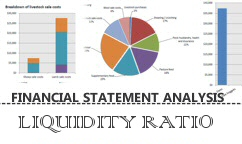Financial Statement Analysis - Liquidity Ratios

In analyzing Financial Statements for the purpose of granting credit, ratios can be broadly classified into three categories.

LIQUIDITY RATIOS

Liquidity Ratios are ratios that come-off the the Balance Sheet and hence measure the liquidity of the company as on a particular day i.e the day that the Balance Sheet was prepared. These ratios are important in measuring the ability of a company to meet both its short term and long term obligations.

##### 01 FIRST LIQUIDITY RATIO

Current Ratio: This ratio is obtained by dividing the 'Total Current Assets' of a company by its 'Total Current Liabilities'. The ratio is regarded as a test of liquidity for a company. It expresses the 'working capital' relationship of current assets available to meet the company's current obligations.

The Formula:

Current Ratio = Total Current Assets/ Total Current Liabilities

An example from our Balance sheet:

Current Ratio = \$261,050 / \$176,522

Current Ratio = 1.48

The Interpretation:

Lumber & Building Supply Company has \$1.48 of Current Assets to meet \$1.00 of its Current Liability

Review the Industry Norms and Ratios for this ratio to compare and see if they are above below or equal to the others in the same industry.

To use the Current Ratio Calculator Click here

##### 02 SECOND LIQUIDITY RATIO

Quick Ratio: This ratio is obtained by dividing the 'Total Quick Assets' of a company by its 'Total Current Liabilities'. Sometimes a company could be carrying heavy inventory as part of its current assets, which might be obsolete or slow moving. Thus eliminating inventory from current assets and then doing the liquidity test is measured by this ratio. The ratio is regarded as an acid test of liquidity for a company. It expresses the true 'working capital' relationship of its cash, accounts receivables, prepaids and notes receivables available to meet the company's current obligations.

The Formula:

Quick Ratio = Total Quick Assets/ Total Current Liabilities

Quick Assets = Total Current Assets (minus) Inventory

An example from our Balance sheet:

Quick Ratio = \$261,050- \$156,822 / \$176,522

Quick Ratio = \$104,228 / \$176,522

Quick Ratio = 0.59

The Interpretation:

Lumber & Building Supply Company has \$0.59 cents of Quick Assets to meet \$1.00 of its Current Liability

Review the Industry Norms and Ratios for this ratio to compare and see if they are above below or equal to the others in the same industry.

To use the Quick Ratio Calculator Click here

##### 03 THIRD LIQUIDITY RATIO

Debt to Equity Ratio: This ratio is obtained by dividing the 'Total Liability or Debt ' of a company by its 'Owners Equity a.k.a Net Worth'. The ratio measures how the company is leveraging its debt against the capital employed by its owners. If the liabilities exceed the net worth then in that case the creditors have more stake than the shareowners.

The Formula:

Debt to Equity Ratio = Total Liabilities / Owners Equity or Net Worth

An example from our Balance sheet:

Debt to Equity Ratio = \$186,522 / \$133,522

Debt to Equity Ratio = 1.40

The Interpretation:

Lumber & Building Supply Company has \$1.40 cents of Debt and only \$1.00 in Equity to meet this obligation.

Review the Industry Norms and Ratios for this ratio to compare and see if they are above below or equal to the others in the same industry.

To use the Debt to Equity Ratio Calculator Click here

Related Articles: